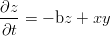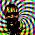## Friday, January 15, 2010

### Lorenz 63 Ensemble

Dan Hughes has started an ambitious series of posts about formally verifying (using the method of manufactured solutions (MMS)) a range of chaotic ordinary differential equations (ODE) systems.
One of the classic ones he’s looking at is Lorenz’s three-dimensional toy system from 1963:(1)(2)(3)
where the system exhibits chaotic behaviour depending on the choice of parameter values (Ra = 28, Pr = 10.0, b = 8∕3 will be used throughout).
The first thing to do is define our governing equations in a computer algebra system (I like Maxima) because that makes writing bug free numerical software and complicated MMS forcing functions much easier.
unknowns : [x,y,z] $depends(unknowns, t)$

/* define the governing equations: */
expr_1 : -Pr * x + Pr * y - diff(x,t) $eqn_1 : solve(expr_1, diff(x,t))$
expr_2 : -y + Ra * x - x * z - diff(y,t) $eqn_2 : solve(expr_2, diff(y,t))$
expr_3 : -* z + x * y - diff(z,t) $eqn_3 : solve(expr_3, diff(z,t))$
Then we need to define our discrete approximation to the governing equations (I’ll skip the MMS stuff for now)
/* elements of the Butcher tableau: */
A : matrix([1/4, -1/4],
[1/4, 5/12]) $bT : matrix([1/4, 3/4])$
c : matrix(, [2/3]) $/* identity matrix for doing Kronecker products, dimension depends on the size of the system you are trying to solve */ sys_I : diagmatrix(3, 1)$

K : transpose([k, k, k, k, k, k]) $Xn : transpose([x[n],y[n],z[n],x[n],y[n],z[n]])$
tn : transpose([t[n], t[n], t[n], t[n], t[n], t[n]]) $F : transpose([f, f, f, f, f, f])$

A_rk : kronecker_product(A, sys_I) $bT_rk : kronecker_product(bT, sys_I)$
hc_rk : kronecker_product(t[n] + h*c, sys_I) $/* argument to our system right-hand-side operator: */ nonlin_arg : Xn + h * A_rk . K$
/* set up the two-stage system: */
rk_sys : zeromatrix(6,1) $q_sys : zeromatrix(6,1)$
rk_sys[1,1] : k - subst(x=nonlin_arg[1,1], subst(y=nonlin_arg[2,1],
subst(z=nonlin_arg[3,1], rhs(eqn_1)))) $rk_sys[2,1] : k - subst(x=nonlin_arg[1,1], subst(y=nonlin_arg[2,1], subst(z=nonlin_arg[3,1], rhs(eqn_2))))$
rk_sys[3,1] : k - subst(x=nonlin_arg[1,1], subst(y=nonlin_arg[2,1],
subst(z=nonlin_arg[3,1], rhs(eqn_3)))) $rk_sys[4,1] : k - subst(x=nonlin_arg[4,1], subst(y=nonlin_arg[5,1], subst(z=nonlin_arg[6,1], rhs(eqn_1))))$
rk_sys[5,1] : k - subst(x=nonlin_arg[4,1], subst(y=nonlin_arg[5,1],
subst(z=nonlin_arg[6,1], rhs(eqn_2)))) $rk_sys[6,1] : k - subst(x=nonlin_arg[4,1], subst(y=nonlin_arg[5,1], subst(z=nonlin_arg[6,1], rhs(eqn_3))))$
This is basically the same approached that I applied to the simple harmonic oscillator, but this time our system is non-linear, so we can’t invert the time-integration operator directly. We need to use an iterative Newton’s method to solve for each time-step, for which we need to define a Jacobian:
/* calculate the system Jacobian for use in a Newtons method: */
J : zeromatrix(6,6) $for j : 1 thru 6 do for i : 1 thru 6 do ( J[i,j] : diff(rk_sys[i,1], k[j]) ) )$

time_update : factor(h* bT_rk . K) 
The really nice thing about putting in a bit of work up-front with the symbol manipulation software is you can just dump the resulting expressions to compile-able code:
load(f90) $file_output_append : false$
fname : ”rhs.f90” $with_stdout(fname, print(”! generated by lorentz63_mms.mac”))$
file_output_append : true $with_stdout(fname, f90(f = rk_sys[1,1]))$
/* ...much more boring file IO follows... */
With all of our useful expressions safely (and correctly) in Fortran, we can compile with F2py and play with them in Python. Integrating the system with a third-order implicit Runge-Kutta method gives the following time-step convergence behaviour:

 Figure 1: Time-step convergence for Lorenz ’63 system with 2-stage Radau-IA RK scheme

Figure 1 shows that as the time-step is decreased the trajectory stays with the ’pack’ for longer and longer times, but when the answers do diverge significantly the change is quite abrupt.
The most practically interesting thing with chaotic systems is the high sensitivity to initial conditions. This sensitivity competes with our uncertain knowledge of the true state of things in determining the limit on our ability to predict the trajectory of (some) dynamic systems (even if our knowledge were perfect, the finite precision of our machines still causes us significant trouble). Part of the way we deal with uncertainty is to do averaging over ensembles. To illustrate this idea I’ll start 7 realizations of the previous calculation, each adjusted by a small amount (on the order of the floating point round-off error for my machine).
nt = 400000
T = 40.0 # integration period
Pr = 10.0
Ra = 28.0
b = 8.0 / 3.0
# knobs for the Newtons method:
tol = 1e-12
maxits = 20
nic = 7 # number of different ICs in our ensemble
h = T / float(nt)
x = sp.zeros((nt,nic), dtype=float, order=’F’)
y = sp.zeros((nt,nic), dtype=float, order=’F’)
z = sp.zeros((nt,nic), dtype=float, order=’F’)
t = sp.linspace(0.0, T, nt)
# some perturbations:
eps = 1e-16 * sp.array([0, 1, -1, 2, -2, 3, -3])
for i in xrange(nic):
x[i] = 1.0 + eps[i]
y[i] = -1.0 + eps[i]
z[i] = 10.0 + eps[i]
I’ll then calculate the pair-wise difference between each trajectory (thats 7 choose 2 or 21 differences). The combination function from the itertools module comes in handy here
from itertools import combinations
from scipy.misc import comb

# calculate our ensemble of trajectories:
for i in xrange(nic):
L63.time_loop(x[:,i],y[:,i],z[:,i],t,Ra,Pr,b,h,tol,maxits)

# calculate all the pair-wise differences between trajectories (nic
# choose 2 of em):
npairs = comb(nic, 2, exact=1)
xdiffs = sp.zeros((nt,npairs), dtype=float, order=’F’)
ydiffs = sp.zeros((nt,npairs), dtype=float, order=’F’)
zdiffs = sp.zeros((nt,npairs), dtype=float, order=’F’)
for i, pair in enumerate(combinations(xrange(nic), 2)):
xdiffs[:,i] = x[:,pair] - x[:,pair]
ydiffs[:,i] = y[:,pair] - y[:,pair]
zdiffs[:,i] = z[:,pair] - z[:,pair]
Here’s the resulting differences:

 Figure 2: Differences between members of the ensemble

You can see that things go along well in the early time, but eventually the differences between ensemble members grows suddenly to be on the same order of magnitude as the things we care about predicting.

#### 1 comment:

1.If you are interested in ensemble forecasting there's a nice article by Boyd, which presents his 'laws':

Logarithmic Law of Arithmurgy
Insight grows logarithmically with the number of floating-point operations.
Extended Moore's Law
Computational power and scientific data as measured by operations and bytes, both grow exponentially with time.
Corollary: Linearity of Progress with Time
Knowledge, as opposed to mere facts or data, grows linearly with time.